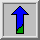# Pixels

In order for any digital computer processing to be carried out on an image, it must first be stored within the computer in a suitable form that can be manipulated by a computer program. The most practical way of doing this is to divide the image up into a collection of discrete (and usually small) cells, which are known as pixels. Most commonly, the image is divided up into a rectangular grid of pixels, so that each pixel is itself a small rectangle. Once this has been done, each pixel is given a pixel value that represents the color of that pixel. It is assumed that the whole pixel is the same color, and so any color variation that did exist within the area of the pixel before the image was discretized is lost. However, if the area of each pixel is very small, then the discrete nature of the image is often not visible to the human eye.

Other pixel shapes and formations can be used, most notably the hexagonal grid, in which each pixel is a small hexagon. This has some advantages in image processing, including the fact that pixel connectivity is less ambiguously defined than with a square grid, but hexagonal grids are not widely used. Part of the reason is that many image capture systems (e.g. most CCD cameras and scanners) intrinsically discretize the captured image into a rectangular grid in the first instance.